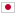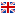Contest Duration: - (local time) (300 minutes)
E - Tree Separator/Time Limit: 2 sec / Memory Limit: 512 MB

### Problem Statement

You are given a tree $T$ and an integer $K$. You can choose arbitrary distinct two vertices $u$ and $v$ on $T$. Let $P$ be the simple path between $u$ and $v$. Then, remove vertices in $P$, and edges such that one or both of its end vertices is in $P$ from $T$. Your task is to choose $u$ and $v$ to maximize the number of connected components with $K$ or more vertices of $T$ after that operation.

### Input

The input consists of a single test case formatted as follows.

$N \ K$ $u_1 \ v_1$ $\vdots$ $u_{N-1} \ v_{N-1}$

The first line consists of two integers $N, K \ (2 \le N \le 100{,}000, 1 \le K \le N)$. The following $N-1$ lines represent the information of edges. The $(i+1)$-th line consists of two integers $u_i, v_i \ (1 \le u_i, v_i \le N \text{ and } u_i \ne v_i \text{ for each } i)$. Each $\{u_i, v_i\}$ is an edge of $T$. It's guaranteed that these edges form a tree.

### Output

Print the maximum number of connected components with $K$ or more vertices in one line.

### Sample Input 1

2 1
1 2


### Output for Sample Input 1

0


### Sample Input 2

7 3
1 2
2 3
3 4
4 5
5 6
6 7


### Output for Sample Input 2

1


### Sample Input 3

12 2
1 2
2 3
3 4
4 5
3 6
6 7
7 8
8 9
6 10
10 11
11 12


### Output for Sample Input 3

4


### Sample Input 4

3 1
1 2
2 3


### Output for Sample Input 4

1


### Sample Input 5

3 2
1 2
2 3


### Output for Sample Input 5

0


### Sample Input 6

9 3
1 2
1 3
1 4
4 5
4 6
4 7
7 8
7 9


### Output for Sample Input 6

2